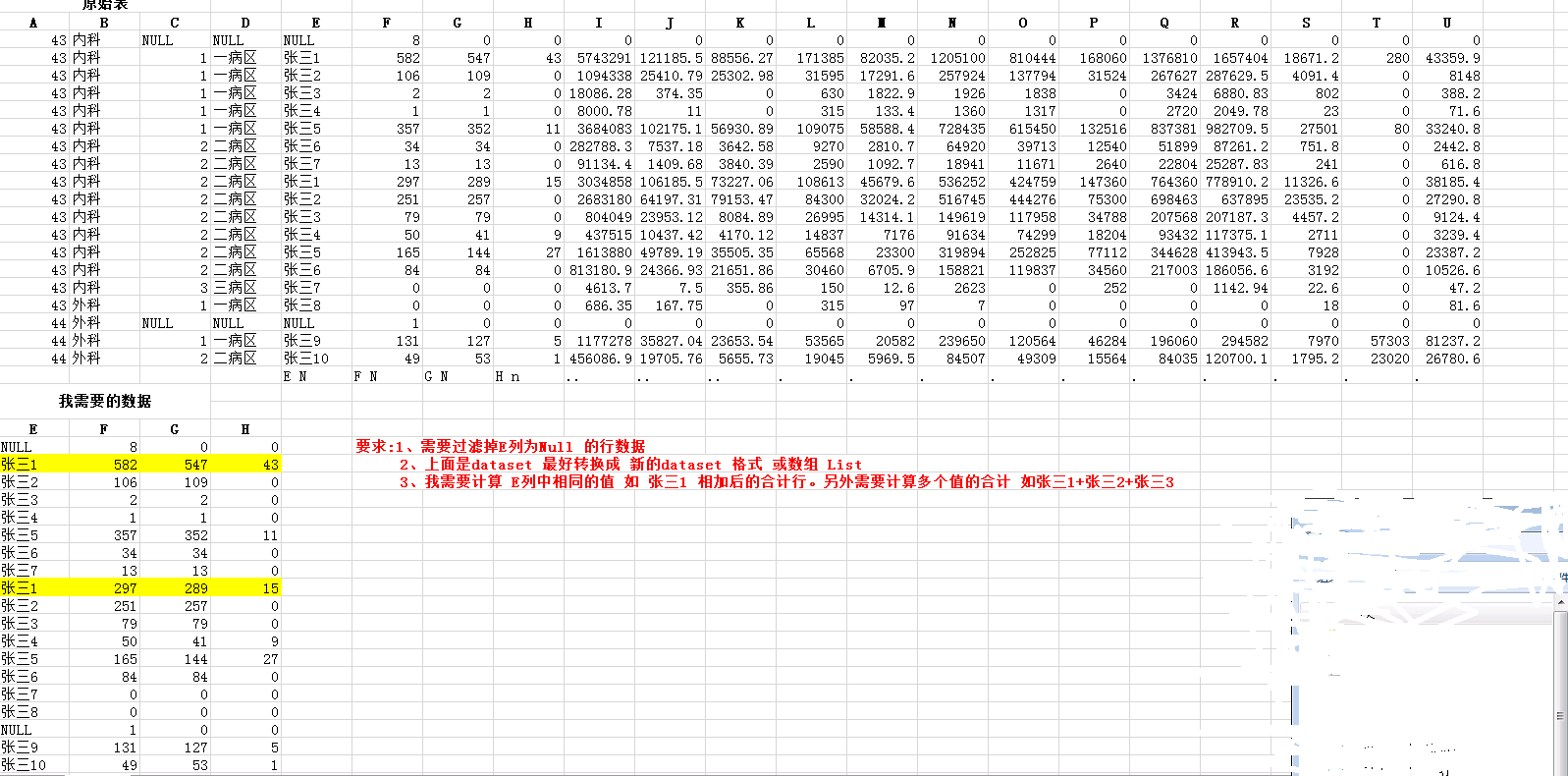2、上面是dataset 最好转换成 新的dataset 格式 或数组 List

3、我需要计算 E列中相同的值 如 张三1 相加后的合计行。另外需要计算多个值的合计 如张三1+张三2+张三3

1个回答

`````` 用linq

var query = dataset.Tables.Rows.Cast<DataRow>().GroupBy(x =>x["e"].ToString())
.Select(x => new { E = x.Key, F = x.Select(y => int.Parse(y["F"])).Sum(), G = ... G = ... });
``````